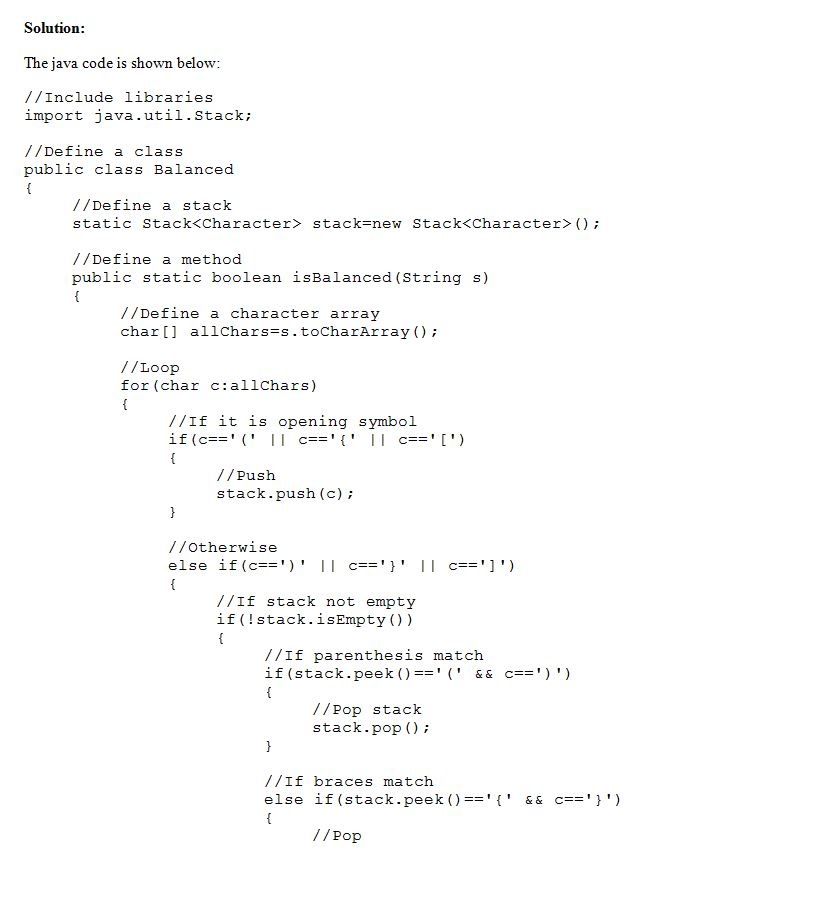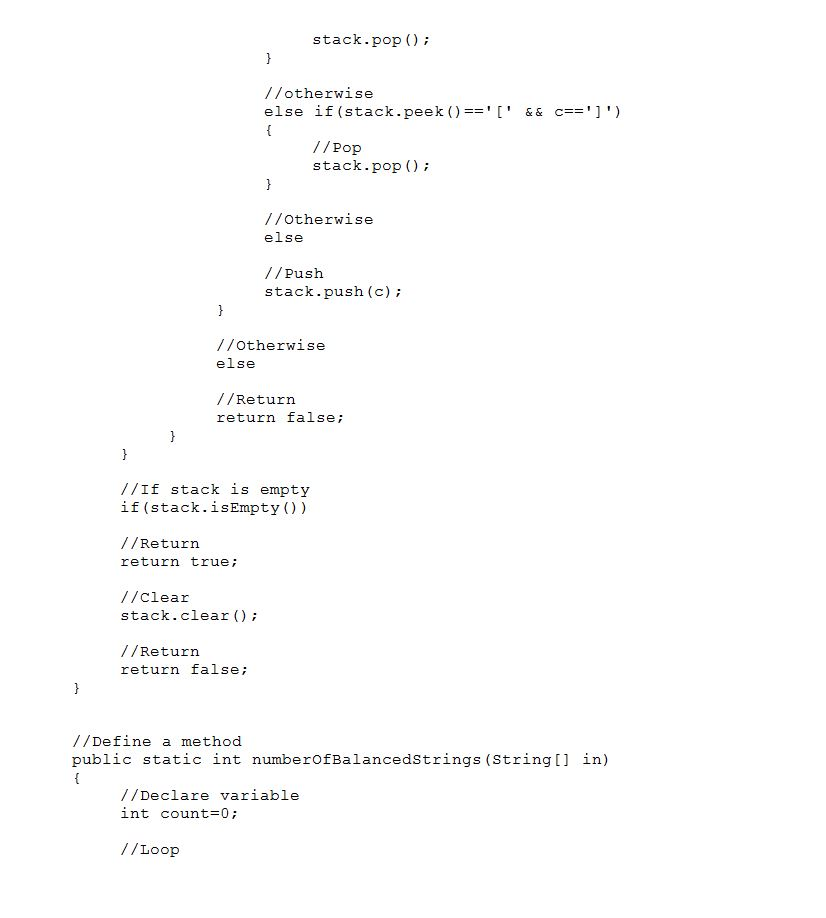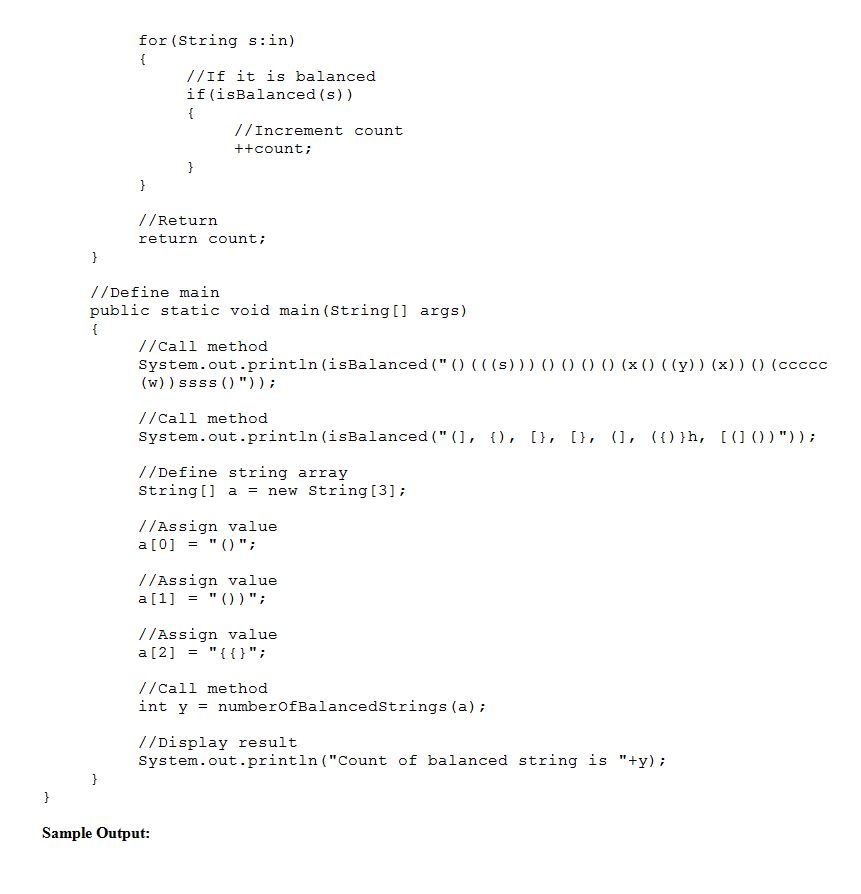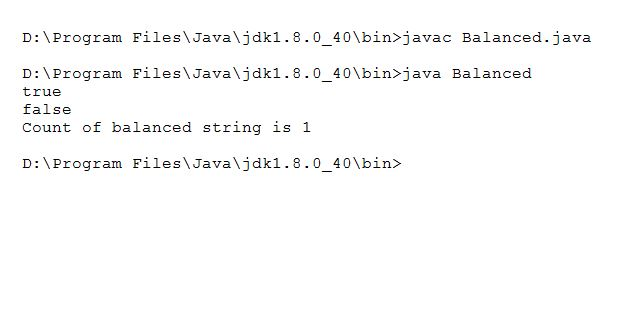# Question & Answer: need help with this code: have to complete the balanced code adding at the end of the…..

need help with this code: have to complete the balanced code adding at the end of the code: with providing a testing code for the code.

A stack is a data structure where data is accessed using the LIFO (last in first out) principle. In this problem, you will use a stack to check whether a string has balanced parentheses (, ) and brackets {, }, [, ], or not.

Don't use plagiarized sources. Get Your Custom Essay on
Question & Answer: need help with this code: have to complete the balanced code adding at the end of the…..
GET AN ESSAY WRITTEN FOR YOU FROM AS LOW AS \$13/PAGE

A string that has balanced parentheses and brackets will be said to be balanced. Any character that is not one of (, ), [, ], {, or } is not important when deciding if a string is balanced and can be ignored.

We will define balanced as follows. A string str is balanced

if str does not contain a parenthesis or bracket symbol, or

str consists of a balanced string surrounded by opening and closing parentheses or matching brackets. That is, str is (b), {b} or [b], where b is any balanced string, or

if str is the concatenation of any two balanced strings. That is, str is bc, where b and c are any balanced strings.

You will complete the provided Balanced class that has two static methods isBalanced(String) and numberOfBalancedStrings(String[]).

Your isBalanced method must use the java.util.Stack class (in a way that solves the problem) to receive any grades for this problem.

http://docs.oracle.com/javase/8/docs/api/java/util/Stack.html

Examples

The following strings have balanced parentheses

(), ()()

cat, c(at), (hello)(kitty)

if( ((x-y) < 4) || (x > 12))

()(((s)))()()()()(x()((y))(x))()(ccccc(w))ssss()

The following strings do not have balances parentheses

), )(a), )a(

The following strings have balanced parentheses and brackets

a, [], {}, [()], []{}({[{}]}),

for(int i=0; i<12; i+=1){x[i]+=f(1);}

The following strings do not have balanced parentheses and brackets

(], {), [}, [}, (], ({)}h, [(]())

for(int i=0; i<12; i+=1){

Note: You will receive partial marks if you code only works for parentheses (and not brackets).

_________________________________

 import java.util.Stack; public class Balanced{ /** Checks if a string is balanced or not. @param s is a non-null string @return true of the input s is balanced and false otherwise. **/ public static boolean isBalanced(String s){ return false; } /** Counts the number of balanced strings in the input array. @param in is a non-null array of strings @return the number of strings in the input in that are balanced. **/ public static int numberOfBalancedStrings(String[] in){ return -1; } }Code:

//Include libraries

import java.util.Stack;

//Define a class

public class Balanced

{

//Define a stack

static Stack<Character> stack=new Stack<Character>();

//Define a method

public static boolean isBalanced(String s)

{

//Define a character array

char[] allChars=s.toCharArray();

//Loop

for(char c:allChars)

{

//If it is opening symbol

if(c=='(‘ || c=='{‘ || c=='[‘)

{

//Push

stack.push(c);

}

//Otherwise

else if(c==’)’ || c==’}’ || c==’]’)

{

//If stack not empty

if(!stack.isEmpty())

{

//If parenthesis match

if(stack.peek()=='(‘ && c==’)’)

{

//Pop stack

stack.pop();

}

//If braces match

else if(stack.peek()=='{‘ && c==’}’)

{

//Pop

stack.pop();

}

//otherwise

else if(stack.peek()=='[‘ && c==’]’)

{

//Pop

stack.pop();

}

//Otherwise

else

//Push

stack.push(c);

}

//Otherwise

else

//Return

return false;

}

}

//If stack is empty

if(stack.isEmpty())

//Return

return true;

//Clear

stack.clear();

//Return

return false;

}

//Define a method

public static int numberOfBalancedStrings(String[] in)

{

//Declare variable

int count=0;

//Loop

for(String s:in)

{

//If it is balanced

if(isBalanced(s))

{

//Increment count

++count;

}

}

//Return

return count;

}

//Define main

public static void main(String[] args)

{

//Call method

System.out.println(isBalanced(“()(((s)))()()()()(x()((y))(x))()(ccccc(w))ssss()”));

//Call method

System.out.println(isBalanced(“(], {), [}, [}, (], ({)}h, [(]())”));

//Define string array

String[] a = new String;

//Assign value

a = “()”;

//Assign value

a = “())”;

//Assign value

a = “{{}”;

//Call method

int y = numberOfBalancedStrings(a);

//Display result

System.out.println(“Count of balanced string is “+y);

}

}